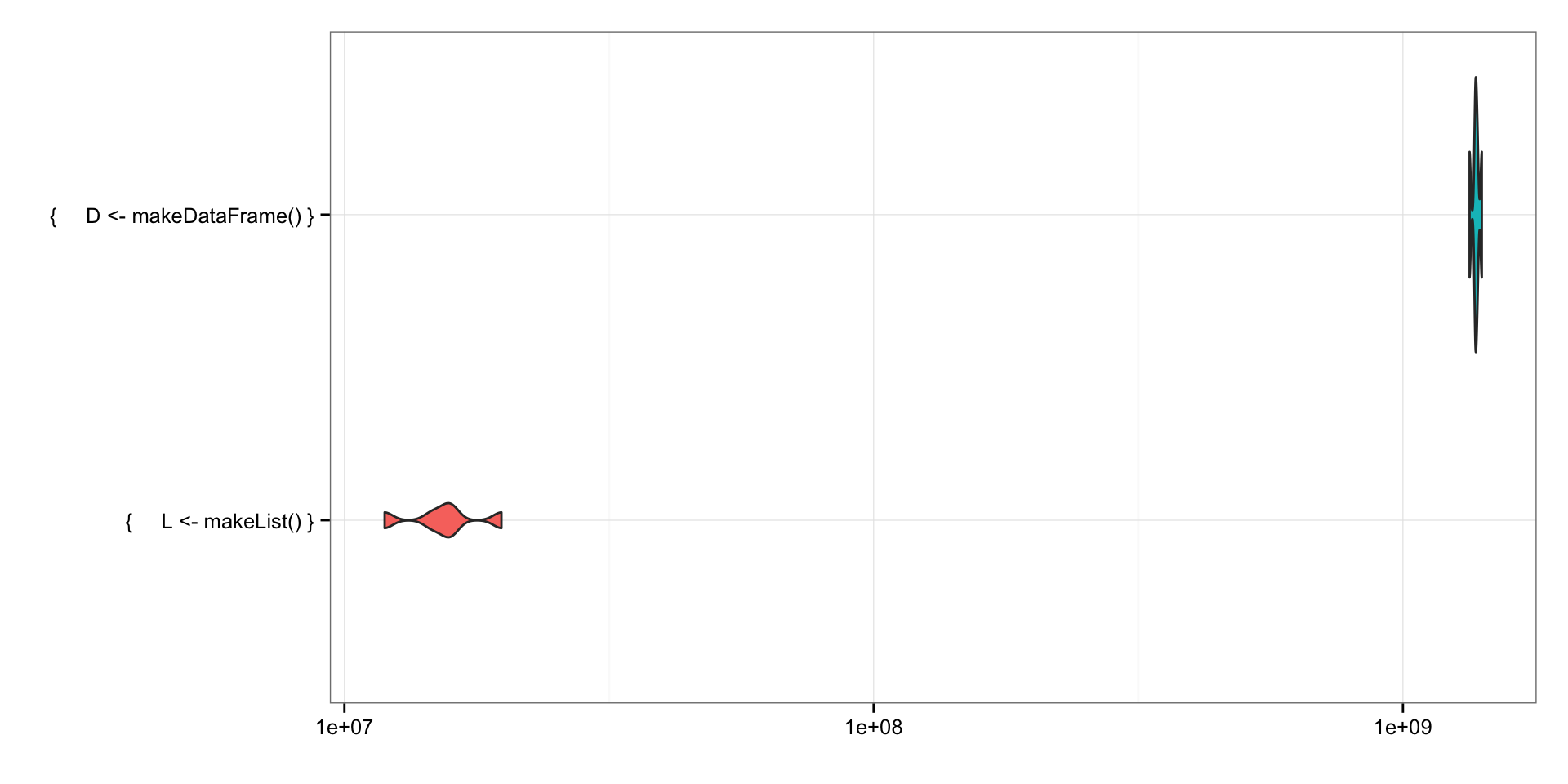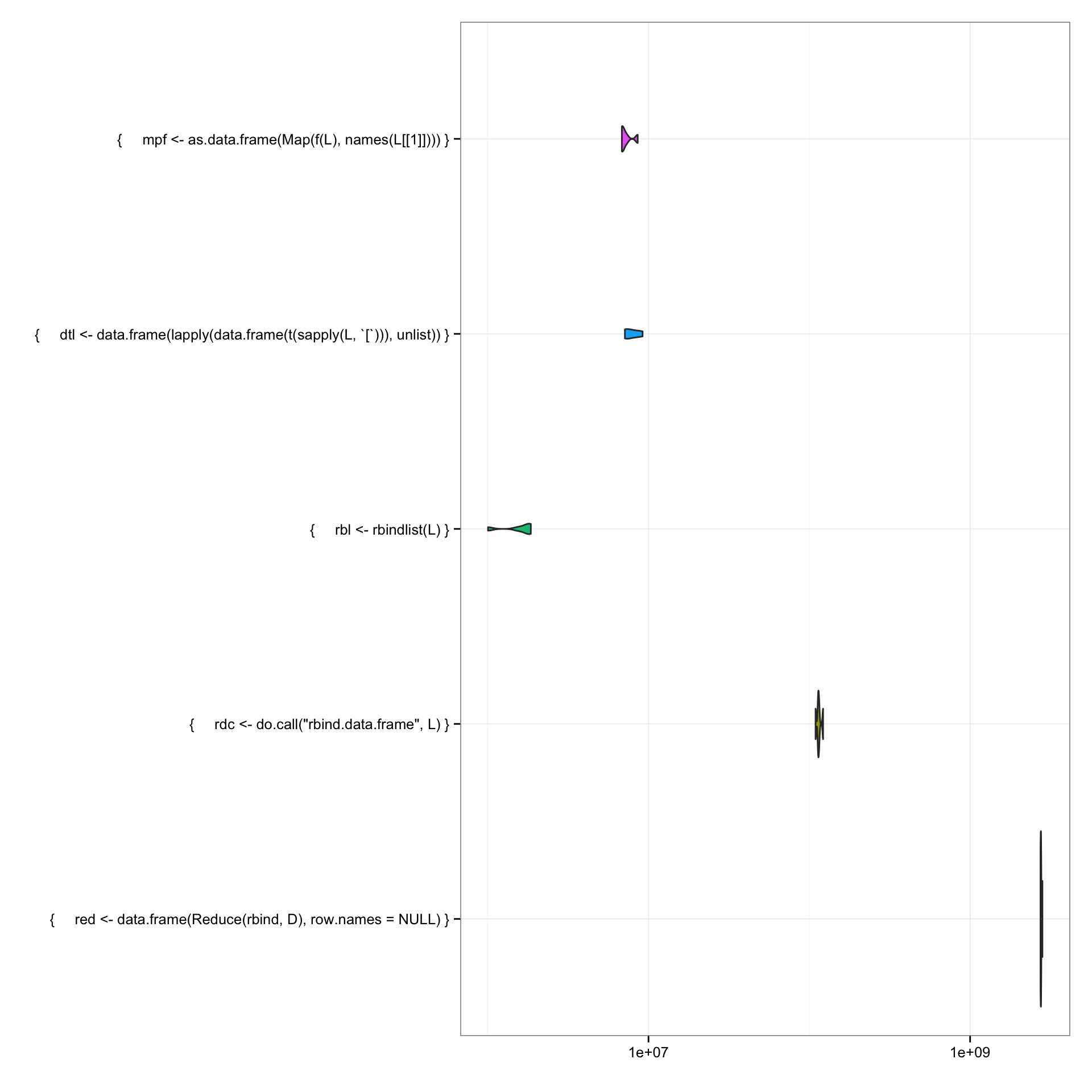There are many times when it becomes necessary to iteratively process data and build a data frame up from scratch. There are many ways to do this in R, some faster than others and some more limited than others (i.e. they return data frames that are either all character or embed list structures into them).

The following document shows five ways to build a data frame out of a list of mixed character, numeric and logical data.

First, we make our list structures, one is a list of lists and the other is a list of data frames. The latter is necessary when using the Reduce method.

We will “cheat” and also use data.table since it provides the rbindlist function.

First, we setup our environment and build our list structures and do some timings of just that:

library(data.table)
library(microbenchmark)
library(ggplot2)

makeList <- function() {
lapply(rep(LETTERS, 100), function(x) {
list(letter=x, another_letter=x, yet_another_letter=x,
a_numeric=match(x, LETTERS), a_logical=x %in% c("A", "E", "I", "O", "U"))
})
}

makeDataFrame <- function() {
lapply(rep(LETTERS, 100), function(x) {
data.frame(letter=x, another_letter=x, yet_another_letter=x,
a_numeric=match(x, LETTERS), a_logical=x %in% c("A", "E", "I", "O", "U"))
})
}

# need this for Map
f = function(x) function(i) unlist(lapply(x, [[, i), use.names=FALSE)

# Make our sample list of lists; note that even this step takes less time than
# doing it with a data frame
structures_bench <- microbenchmark({L <- makeList()},
{D <- makeDataFrame()}, times=5)

print(structures_bench, unit="s", order="median")
## Unit: seconds
##                          expr     min      lq  median      uq     max
##       {     L <- makeList() } 0.01188 0.01469 0.01577 0.01586 0.01986
##  {     D <- makeDataFrame() } 1.33608 1.36953 1.37335 1.38284 1.40987
##  neval
##      5
##      5(plots are in nanoseconds and on a log scale, tables are ordered by the median column)

From that alone, we see we’ll get better speed out of not calling data.frame repeatedly.

Now we’ll run five different conversion functions:

• the first uses Reduce to perform an rbind on pairs of elements from L.
• the do.call method passes all of the elements as a call to the rbind.data.frame function.
• rbindlist is pretty self-explanatory.
• the fourth does a max transposition of the list of lists, converts it to a data frame of lists (ugh) then re-builds the columns as intended
• the last used Map with a helper function (defined above) that returns a function that extracts the i’th element of x
list_to_df_bench <- microbenchmark(
{red <- data.frame(Reduce(rbind, D), row.names=NULL)},
{rdc <- do.call("rbind.data.frame", L)},
{rbl <- rbindlist(L)},
{dtl <- data.frame(lapply(data.frame(t(sapply(L, [))), unlist))},
{mpf <- as.data.frame(Map(f(L), names(L[])))}, times=5)

print(list_to_df_bench, unit="s", order="median")
## Unit: seconds
##                                                                      expr
##                                               {     rbl <- rbindlist(L) }
##                    {     mpf <- as.data.frame(Map(f(L), names(L[]))) }
##  {     dtl <- data.frame(lapply(data.frame(t(sapply(L, [))), unlist)) }
##                             {     rdc <- do.call("rbind.data.frame", L) }
##             {     red <- data.frame(Reduce(rbind, D), row.names = NULL) }
##       min       lq   median       uq      max neval
##  0.001000 0.001556 0.001796 0.001796 0.001864     5
##  0.006823 0.006863 0.006900 0.007294 0.008582     5
##  0.007121 0.007122 0.007683 0.008372 0.009209     5
##  0.109344 0.113362 0.114015 0.115235 0.121870     5
##  2.739949 2.747488 2.759248 2.765681 2.816467     5Finally, we’ll inspect each data structure to show they are really proper data.frames or data.tables:

str(red)
## 'data.frame':    2600 obs. of  5 variables:
##  $letter : Factor w/ 26 levels "A","B","C","D",..: 1 2 3 4 5 6 7 8 9 10 ... ##$ another_letter    : Factor w/ 26 levels "A","B","C","D",..: 1 2 3 4 5 6 7 8 9 10 ...
##  $yet_another_letter: Factor w/ 26 levels "A","B","C","D",..: 1 2 3 4 5 6 7 8 9 10 ... ##$ a_numeric         : int  1 2 3 4 5 6 7 8 9 10 ...
##  $a_logical : logi TRUE FALSE FALSE FALSE TRUE FALSE ... str(rbl) ## Classes 'data.table' and 'data.frame': 2600 obs. of 5 variables: ##$ letter            : chr  "A" "B" "C" "D" ...
##  $another_letter : chr "A" "B" "C" "D" ... ##$ yet_another_letter: chr  "A" "B" "C" "D" ...
##  $a_numeric : int 1 2 3 4 5 6 7 8 9 10 ... ##$ a_logical         : logi  TRUE FALSE FALSE FALSE TRUE FALSE ...
##  - attr(*, ".internal.selfref")=<externalptr>
str(rdc)
## 'data.frame':    2600 obs. of  5 variables:
##  $letter : Factor w/ 26 levels "A","B","C","D",..: 1 2 3 4 5 6 7 8 9 10 ... ##$ another_letter    : Factor w/ 26 levels "A","B","C","D",..: 1 2 3 4 5 6 7 8 9 10 ...
##  $yet_another_letter: Factor w/ 26 levels "A","B","C","D",..: 1 2 3 4 5 6 7 8 9 10 ... ##$ a_numeric         : int  1 2 3 4 5 6 7 8 9 10 ...
##  $a_logical : logi TRUE FALSE FALSE FALSE TRUE FALSE ... str(dtl) ## 'data.frame': 2600 obs. of 5 variables: ##$ letter            : Factor w/ 26 levels "A","B","C","D",..: 1 2 3 4 5 6 7 8 9 10 ...
##  $another_letter : Factor w/ 26 levels "A","B","C","D",..: 1 2 3 4 5 6 7 8 9 10 ... ##$ yet_another_letter: Factor w/ 26 levels "A","B","C","D",..: 1 2 3 4 5 6 7 8 9 10 ...
##  $a_numeric : int 1 2 3 4 5 6 7 8 9 10 ... ##$ a_logical         : logi  TRUE FALSE FALSE FALSE TRUE FALSE ...
str(mpf)
## 'data.frame':    2600 obs. of  5 variables:
##  $letter : Factor w/ 26 levels "A","B","C","D",..: 1 2 3 4 5 6 7 8 9 10 ... ##$ another_letter    : Factor w/ 26 levels "A","B","C","D",..: 1 2 3 4 5 6 7 8 9 10 ...
##  $yet_another_letter: Factor w/ 26 levels "A","B","C","D",..: 1 2 3 4 5 6 7 8 9 10 ... ##$ a_numeric         : int  1 2 3 4 5 6 7 8 9 10 ...
##  \$ a_logical         : logi  TRUE FALSE FALSE FALSE TRUE FALSE ...